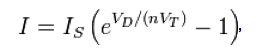# numerical solution for any semiconductor device

Status
Not open for further replies.

#### khos

##### BannedHow can I make a simulator for PN junctions, so we will need to solve some differential equations numerically to simulate the device by using (FENICS/DOLFIN) and used for solving such equations in C++ or python ????????????

##### Super Moderator
Staff memberThere is the diode equation. It may or may not suit your purpose.More can be found at:

https://en.wikipedia.org/wiki/Diode_modelling#Shockley_diode_model

--------------------------------------------

If you want a simple V-to-I formula...

I wanted to find a mathematical equation to model a diode for purposes of simulation.

I performed many tests on diodes with my meter, and then plotted the data, and tried to fit an equation to the graph.

I came up with:

A = ( V x 1.25 ) ^ 20

This is only for a typical silicon diode. It will require a different equation to model an LED or a germanium or a schottky or a power diode.

Status
Not open for further replies.